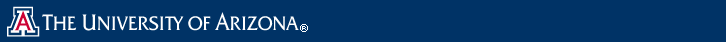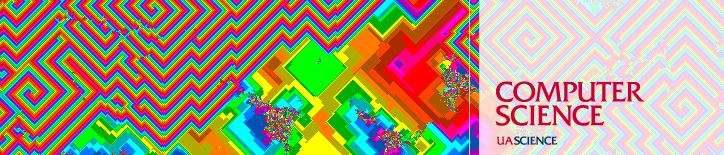Colloquium

 Category Lecture Date Thursday, March 4, 2010 Time 11:00 am Location GS 906 Details Light refreshments - 9th floor Atrium - 10:45 am Speaker Tetsuo Asano Title School of Information Science Affiliation JAIST (Japan Advanced Institute of Science and Technology)

Constant-Work-Space Algorithm for Geometric Problems

Abstract: I will talk about space-efficient algorithms for geometric problems in a restricted computational model called constant work space''
or log-space'' computation. I start with an algorithm for drawing
a Delaunay triangulation of a planar point set and then extend it
to another algorithm for drawing a Voronoi diagram.
There are $\Theta(n \log n)$-time algorithms for those problems in a
standard computational model for $n$ points. Our algorithms run in
$O(n2)$ time using only a constant number of storage cells of
$O(\log n)$ bits in total. Then, I will give a cubic-time algorithm
for computing a Euclidean minimum spanning tree for a point set.
The fourth problem is to find a simple path between two arbitrary
nodes in a tree of $n$ nodes. Our algorithm runs in $O(n^{1+\epsilon})$
time using work space of size $O(1/\epsilon)$ for any small constant
$\epsilon > 0$. Then, I will describe how to compute a shortest
path between two points in a simple $n$-gon. Although the shortest
path problem in general graphs is NL-complete, this constrained
problem can be solved in quadratic time using only constant work
space.

Biography

Dr. Tetsuo Asano was born in Kyoto Prefecture, Japan, in 1949.
He got B.E., M.E., and Ph.D degrees from Osaka University, Japan,
in 1972, 1974, and 1977, respectively. In 1977 he joined
Osaka Electro-Communication University as a lecturer and moved to
JAIST (Japan Advanced Institute of Science and technology) in 1997.
He is now a professor in School of Information Science. He served
as a Presidential Advisor in 1999-2000 and as a senetor in
2001-2003. His research interest includes algorithms and data
structures, especially in computational geometry, combinatorial
optimization, computer graphics, computer vision using geometric
information, and VLSI layout design. He has been serving as editors
of several journals including Discrete and Computational Geometry,
Computational Geometry: Theory and Applications, International
Journal of Computational Geometry and Applications, and Theory of
Computing Sysytems. He has contributed to ACM Annual Symposium on
Computational Geometry as a program committee member in 1990, 1994,
1996, and 2004. He served as a chair of a Special Interest
Group on Algorithms of Information Processing Society of Japan in
1994-1996. He is fellows of Association of Computing Machinery
2001) and Information Processing Soceity of Japan (2004).
Currently he is an advisor to the President of JAIST on education
and will be a director of a new center for education at JAIST.